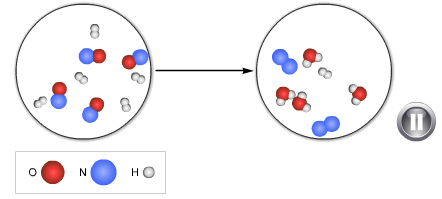# Problem: For the reaction shown above, what is the chemical formula for the limiting reactant? Write the balanced chemical equation for the reaction, using lowest whole-number coefficients.

###### FREE Expert Solution

To determine the limiting reactant, we shall determine which of the reactants is smaller in number/amount.

80% (87 ratings)###### Problem DetailsFor the reaction shown above, what is the chemical formula for the limiting reactant?

Write the balanced chemical equation for the reaction, using lowest whole-number coefficients.